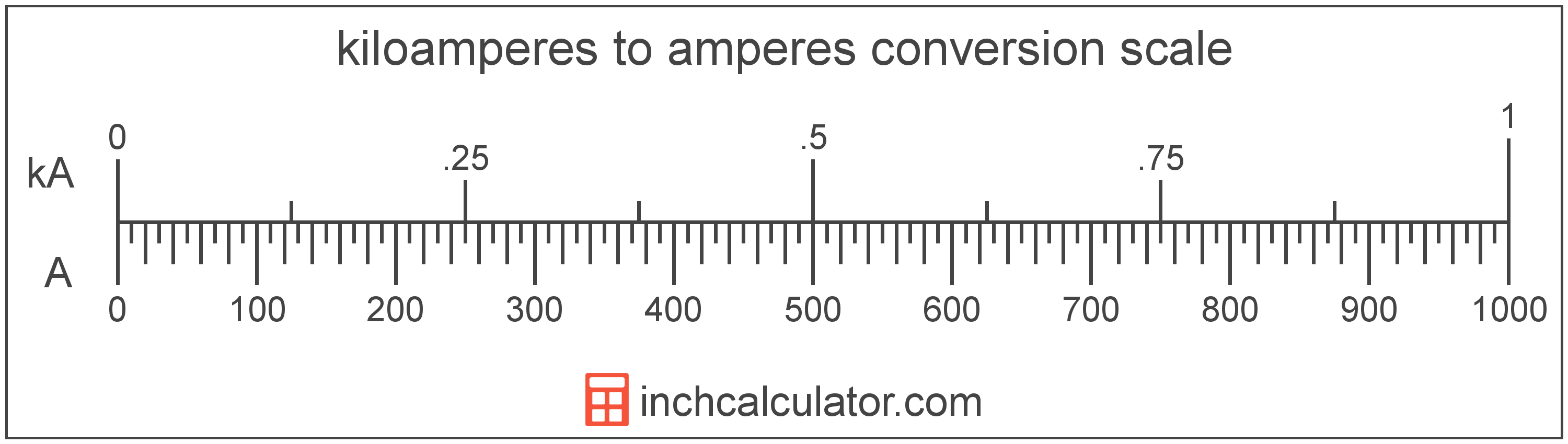# Amperes to Kiloamperes Converter

Enter the electric current in amperes below to get the value converted to kiloamperes.

Results in Kiloamperes:1 A = 0.001 kA

Do you want to convert kiloamperes to amperes?

## How to Convert Amperes to Kiloamperes

To convert a measurement in amperes to a measurement in kiloamperes, divide the electric current by the following conversion ratio: 1,000 amperes/kiloampere.

Since one kiloampere is equal to 1,000 amperes, you can use this simple formula to convert:

kiloamperes = amperes ÷ 1,000

The electric current in kiloamperes is equal to the electric current in amperes divided by 1,000.

For example, here's how to convert 5,000 amperes to kiloamperes using the formula above.
kiloamperes = (5,000 A ÷ 1,000) = 5 kA## What Is an Ampere?

The ampere, commonly referred to as an "amp," is the electrical current constant equal to the flow of one coulomb per second.

The ampere was previously defined as a constant current that when passed through two straight and parallel conductors that are spaced one meter apart, will produce a force equal to 0.0000002 newtons per meter of length.

In 2019, the ampere was redefined as the electric current corresponding to the flow of 1/(1.602 176 634 × 10-19) elementary charges per second.

The ampere is the SI base unit for electric current in the metric system. An ampere is sometimes also referred to as an amp. Amperes can be abbreviated as A; for example, 1 ampere can be written as 1 A.

### Amperage and Ohm's Law

Ohm's Law states the current between two points on a conductor is proportional to the voltage and inversely proportional to the resistance. Using Ohm's Law, it's possible to express the current in amperes as an expression using resistance and voltage.

IA = VV / R

The current in amperes is equal to the potential difference in volts divided by the resistance in ohms.

## What Is a Kiloampere?

One kiloampere is equal to 1,000 amperes, which are the electrical current equal to the flow of one coulomb per second.

The kiloampere is a multiple of the ampere, which is the SI base unit for electric current. In the metric system, "kilo" is the prefix for thousands, or 103. A kiloampere is sometimes also referred to as a kiloamp. Kiloamperes can be abbreviated as kA; for example, 1 kiloampere can be written as 1 kA.

## Ampere to Kiloampere Conversion Table

Table showing various ampere measurements converted to kiloamperes.
Amperes Kiloamperes
1 A 0.001 kA
2 A 0.002 kA
3 A 0.003 kA
4 A 0.004 kA
5 A 0.005 kA
6 A 0.006 kA
7 A 0.007 kA
8 A 0.008 kA
9 A 0.009 kA
10 A 0.01 kA
20 A 0.02 kA
30 A 0.03 kA
40 A 0.04 kA
50 A 0.05 kA
60 A 0.06 kA
70 A 0.07 kA
80 A 0.08 kA
90 A 0.09 kA
100 A 0.1 kA
200 A 0.2 kA
300 A 0.3 kA
400 A 0.4 kA
500 A 0.5 kA
600 A 0.6 kA
700 A 0.7 kA
800 A 0.8 kA
900 A 0.9 kA
1,000 A 1 kA

## References

1. International Bureau of Weights and Measures, The International System of Units, 9th Edition, 2019, https://www.bipm.org/documents/20126/41483022/SI-Brochure-9-EN.pdf